# pdeCoefficients

Extract coefficients of partial differential equation

## Syntax

``coeffs = pdeCoefficients(pdeeq,u)``
``symCoeffs = pdeCoefficients(pdeeq,u,'Symbolic',true)``

## Description

example

````coeffs = pdeCoefficients(pdeeq,u)` extracts the coefficients of a partial differential equation (PDE) as a structure of double-precision numbers and function handles, which can be used as input of the `specifyCoefficients` function in Partial Differential Equation Toolbox™.`pdeeq` is a scalar PDE or a PDE system in symbolic form that is a function of `u`. The `pdeCoefficients` function converts `pdeeq` into a system of equations of the form $m\frac{{\partial }^{2}u}{\partial {t}^{2}}+d\frac{\partial u}{\partial t}-\nabla ·\left(c\otimes \nabla u\right)+au=f$ and returns the structure `coeffs` that contains the coefficients `m`, `d`, `c`, `a`, and `f`. For more information, see Equations You Can Solve Using PDE Toolbox (Partial Differential Equation Toolbox).If `pdeCoefficients` cannot convert a PDE into the divergence form above, then it issues a warning message and writes all remaining gradients to the `f` coefficient. PDE Toolbox will be unable to solve this type of PDE.```

example

````symCoeffs = pdeCoefficients(pdeeq,u,'Symbolic',true)` returns the PDE coefficients as a structure of symbolic expressions.```

## Examples

collapse all

Create a symbolic PDE that represents the Laplacian of the variable `u(x,y)`.

```syms u(x,y) pdeeq = laplacian(u,[x y]) == -3```
```pdeeq(x, y) =  ```

Extract the coefficients of the PDE.

`coeffs = pdeCoefficients(pdeeq,u)`
```coeffs = struct with fields: a: 0 c: [4x1 double] m: 0 d: 0 f: -3 ```

`pdeCoefficients` converts the symbolic PDE into a scalar PDE equation of the form

`$m\frac{{\partial }^{2}u}{\partial {t}^{2}}+d\frac{\partial u}{\partial t}-\nabla \cdot \left(c\nabla u\right)+au=f$`

and extracts the coefficients `m`, `d`, `c`, `a`, and `f` into the structure `coeffs`. For 2-D systems of $N$ equations, `c` is a tensor with 4${N}^{2}$ elements. For more information, see c Coefficient for specifyCoefficients (Partial Differential Equation Toolbox). In this case, $N=1$, so the coefficient `c` is a 4-by-1 row vector that represents `c`${}_{xx}$, `c`${}_{xy}$, `c`${}_{yx}$, and `c`${}_{yy}$.

`coeffs.c`
```ans = 4×1 -1 0 0 -1 ```

Solve a 2-D homogenous Laplace's equation in the domain bounded by a unit circle. Use the `pdeCoefficients` function to extract the coefficients of the Laplace's equation.

Create the PDE model and include the geometry.

```model = createpde; geometryFromEdges(model,@circleg);```

Plot the geometry and display the edge labels for use in the boundary condition definition.

```figure pdegplot(model,'EdgeLabels','on') axis equal```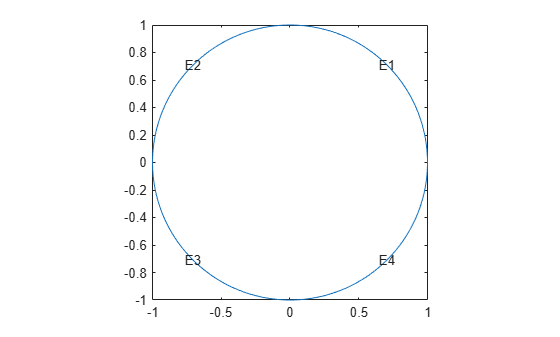Create a symbolic expression `pdeeq` that represents the Laplace's equation.

```syms u(x,y) pdeeq = laplacian(u,[x y])```
```pdeeq(x, y) =  ```

Extract the coefficients of the Laplace's equation.

`coeffs = pdeCoefficients(pdeeq,u)`
```coeffs = struct with fields: a: 0 c: [4x1 double] m: 0 d: 0 f: 0 ```

Specify the coefficients of the PDE model.

```specifyCoefficients(model,'m',coeffs.m,'d',coeffs.d, ... 'c',coeffs.c,'a',coeffs.a,'f',coeffs.f);```

Apply the Dirichlet boundary condition $u\left(x,y\right)={x}^{4}+{y}^{4}$ at all 4 edges that form the circle.

`applyBoundaryCondition(model,'dirichlet','Edge',1:4,'u',@(region,state) region.x.^4 + region.y.^4);`

Generate the default mesh for the geometry.

`generateMesh(model);`

Solve the PDE and plot the solution.

```results = solvepde(model); pdeplot(model,'XYData',results.NodalSolution)```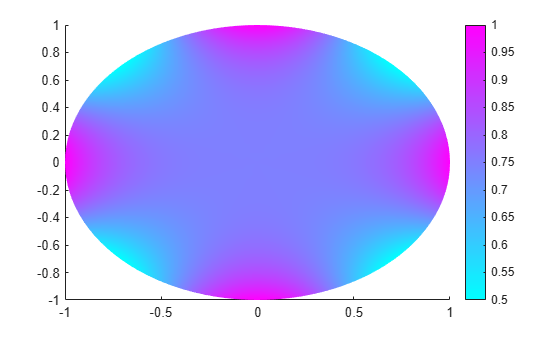Solve a 2-D Poisson's equation in the domain bounded by a unit circle. The divergence form of the PDE has nonconstant `f` coefficient. You can solve the PDE by extracting the coefficients using `pdeCoefficients` and specifying the coefficients in the PDE model using `specifyCoefficients`.

Create the PDE model and include the geometry.

```model = createpde; geometryFromEdges(model,@circleg);```

Create a symbolic expression `pdeeq` that represents Poisson's equation.

```syms u(x,y) pdeeq = diff(u,x,x) + diff(u,y,y) - 1/(x^2 + y^2)```
```pdeeq(x, y) =  ```

Extract the coefficients of the equation.

`coeffs = pdeCoefficients(pdeeq,u)`
```coeffs = struct with fields: a: 0 c: [4x1 double] m: 0 d: 0 f: @makeCoefficient/coefficientFunction ```

The `f` coefficient is not a constant. It is displayed as `@makeCoefficient/coefficientFunction`, which indicates that it has been returned from the workspace of some internal function. To show the formula behind the function handle, use `coeffs.f('show')`.

`coeffs.f('show')`
```ans =  $\frac{1}{{x}^{2}+{y}^{2}}$```

Specify the coefficients of the PDE model.

```specifyCoefficients(model,'m',coeffs.m,'d',coeffs.d, ... 'c',coeffs.c,'a',coeffs.a,'f',coeffs.f);```

Apply the Dirichlet boundary condition $u\left(x,y\right)=0$ at all 4 edges that form the circle.

`applyBoundaryCondition(model,'dirichlet','Edge',1:4,'u',0);`

Generate the default mesh for the geometry.

`generateMesh(model);`

Solve the PDE. Plot the nodal solution using the option `'XYData'` in the `pdeplot` function.

```results = solvepde(model); pdeplot(model,'XYData',results.NodalSolution)```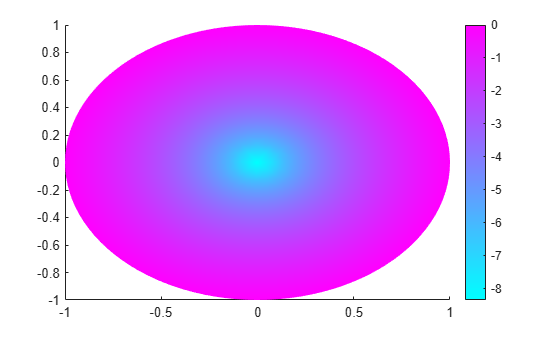Plot the gradient of the solution at the nodal locations using the option `'FlowData'`.

`pdeplot(model,'FlowData',[results.XGradients results.YGradients])`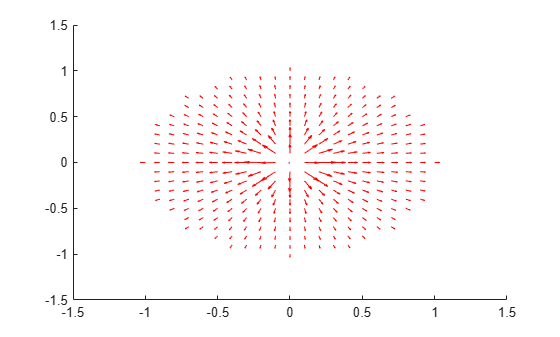Construct a PDE with no divergence form.

```syms u(x,y) pdeeq = diff(u,x,x) + cos(x+y)/4*diff(u,x,y) + 1/2*diff(u,y,y)```
```pdeeq(x, y) =  ```

Extract its coefficients. When `pdeCoefficients` cannot convert a PDE into the divergence form

$m\frac{{\partial }^{2}u}{\partial {t}^{2}}+d\frac{\partial u}{\partial t}-\nabla \cdot \left(c\otimes \nabla u\right)+au=f$,

it issues a warning message, but it still produces output.

`coeffs = pdeCoefficients(pdeeq,u)`
```Warning: After extracting m, d, and c, some gradients remain. Writing all remaining terms to f. ```
```coeffs = struct with fields: a: 0 c: @makeCoefficient/coefficientFunction m: 0 d: 0 f: @makeCoefficient/coefficientFunction ```

To show the function handles in the extracted coefficients `c` and `f`, use the option `'show'`. All remaining gradients in the PDE are written to the `f` coefficient.

`coeffs.c('show')`
```ans =  $\left(\begin{array}{c}-1\\ \frac{1}{8}-\frac{\mathrm{cos}\left(x+y\right)}{4}\\ \frac{1}{8}-\frac{\mathrm{cos}\left(x+y\right)}{4}\\ -\frac{1}{2}\end{array}\right)$```
`coeffs.f('show')`
```ans =  ```

Since the PDE has no divergence form required by the PDE Toolbox, the toolbox will be unable to solve this PDE.

Solve a time-dependent wave equation in the domain bounded by a unit circle. The wave equation is a PDE with a scalar function $u\left(t,x,y\right)$ that depends on time $t$ and coordinates $x$ and $y$.

Create the PDE model and include the geometry.

```model = createpde(1); geometryFromEdges(model,@circleg);```

Create a symbolic PDE that represents the wave equation.

```syms u(t,x,y) pdeeq = diff(u,t,t) - laplacian(u,[x y])```
```pdeeq(t, x, y) =  ```

Extract the coefficients of the PDE.

`coeffs = pdeCoefficients(pdeeq,u)`
```coeffs = struct with fields: a: 0 c: [4x1 double] m: 1 d: 0 f: 0 ```

Specify the coefficients of the PDE model.

```specifyCoefficients(model,'m',coeffs.m,'d',coeffs.d, ... 'c',coeffs.c,'a',coeffs.a,'f',coeffs.f);```

Set the initial conditions of the time-dependent problem on the entire geometry.

`setInitialConditions(model,0,1);`

Apply the Dirichlet boundary condition $u\left(x,y\right)=0$ at all 4 edges that form the circle.

`applyBoundaryCondition(model,'dirichlet','Edge',1:4,'u',0);`

Generate the default mesh for the geometry.

`generateMesh(model);`

Find the solutions of the time-dependent PDE at a time range from 0s to 50s with a 2s interval.

`results = solvepde(model,linspace(0,2,50));`

Plot the solution of the wave equation for each 5s interval.

```figure; for k = 1:10 subplot(5,2,k); pdeplot(model,'XYData',results.NodalSolution(:,k*5)) axis equal end ```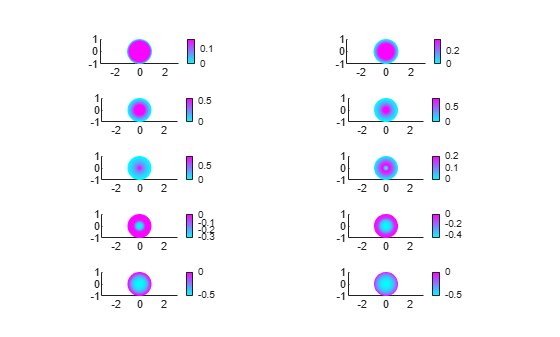Solve a system of two second-order PDEs. You can solve the PDE system by extracting the PDE coefficients symbolically using `pdeCoefficients`, converting the coefficients to double-precision numbers using `pdeCoefficientsToDouble`, and specifying the coefficients in the PDE model using `specifyCoefficients`.

The system of PDEs represents the deflection of a clamped structural plate under a uniform pressure load. The system of PDEs with the dependent variables ${u}_{1}$ and ${u}_{2}$ is given by

$-{\nabla }^{2}{u}_{1}+{u}_{2}=0$,

$-D{\nabla }^{2}{u}_{2}=p$,

where $D$ is the bending stiffness of the plate given by

$D=\frac{E{h}^{3}}{12\left(1-{\nu }^{2}\right)}$,

and $E$ is the modulus of elasticity, $\nu$ is Poisson's ratio, $h$ is the plate thickness, ${u}_{1}$ is the transverse deflection of the plate, and $p$ is the pressure load.

Create a PDE model for the system of two equations.

`model = createpde(2);`

Create a square geometry. Specify the side length of the square. Then include the geometry in the PDE model.

```len = 10.0; gdm = [3 4 0 len len 0 0 0 len len]'; g = decsg(gdm,'S1',('S1')'); geometryFromEdges(model,g);```

Specify the values of the physical parameters of the system. Let the external pressure $p$ be a symbolic variable `pres` that can take any value.

```E = 1.0e6; h_thick = 0.1; nu = 0.3; D = E*h_thick^3/(12*(1 - nu^2)); syms pres```

Declare the PDE system as a system symbolic equations. Extract the coefficients of the PDE and return them in symbolic form.

```syms u1(x,y) u2(x,y) pdeeq = [-laplacian(u1) + u2; -D*laplacian(u2) - pres]; symCoeffs = pdeCoefficients(pdeeq,[u1 u2],'Symbolic',true)```
```symCoeffs = struct with fields: m: [1x1 sym] a: [2x2 sym] c: [4x4 sym] f: [2x1 sym] d: [1x1 sym] ```

Display the coefficients `m`, `a`, `c`, `f`, and `d`.

`structfun(@disp,symCoeffs)`
`$0$`
`$\left(\begin{array}{cc}0& 1\\ 0& 0\end{array}\right)$`
`$\left(\begin{array}{cccc}1& 0& 0& 0\\ 0& 1& 0& 0\\ 0& 0& \frac{25000}{273}& 0\\ 0& 0& 0& \frac{25000}{273}\end{array}\right)$`
`$\left(\begin{array}{c}0\\ \mathrm{pres}\end{array}\right)$`
`$0$`

Substitute a value for `pres` using the `subs` function. Since the outputs of `subs` are symbolic objects, use the `pdeCoefficientsToDouble` function to convert the coefficients to the `double` data type, which makes them valid inputs for the PDE Toolbox.

```symCoeffs = subs(symCoeffs,pres,2); coeffs = pdeCoefficientsToDouble(symCoeffs)```
```coeffs = struct with fields: a: [4x1 double] c: [16x1 double] m: 0 d: 0 f: [2x1 double] ```

Specify the PDE coefficients for the PDE model.

```specifyCoefficients(model,'m',coeffs.m,'d',coeffs.d, ... 'c',coeffs.c,'a',coeffs.a,'f',coeffs.f);```

Specify spring stiffness. Specify boundary conditions by defining distributed springs on all four edges.

```k = 1e7; bOuter = applyBoundaryCondition(model,'neumann','Edge',(1:4), ... 'g',[0 0],'q',[0 0; k 0]);```

Specify the mesh size of the geometry and generate a mesh for the PDE model.

```hmax = len/20; generateMesh(model,'Hmax',hmax);```

Solve the model.

`res = solvepde(model);`

Access the solution at the nodal locations.

`u = res.NodalSolution;`

Plot the transverse deflection of the plate.

```numNodes = size(model.Mesh.Nodes,2); figure; pdeplot(model,'XYData',u(1:numNodes),'contour','on') title 'Transverse Deflection'```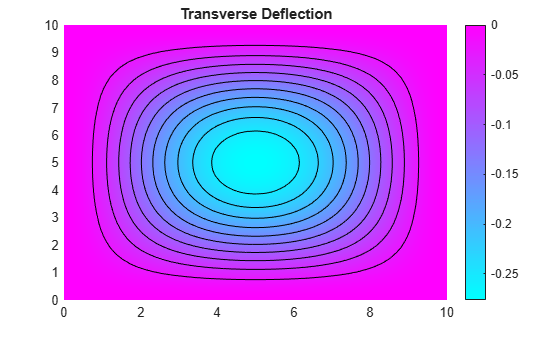Find the transverse deflection at the plate center.

`wMax = min(u(1:numNodes,1))`
```wMax = -0.2763 ```

Compare the result with the deflection at the plate center computed analytically.

```pres = 2; wMax = -.0138*pres*len^4/(E*h_thick^3)```
```wMax = -0.2760 ```

## Input Arguments

collapse all

PDE in symbolic form, specified as a symbolic equation, expression, or a symbolic vector.

Variable of PDE, specified as a symbolic function. `u` must be a stationary or time-dependent variable in two or three dimensions. For example, create the variable `u` using one of these statements:

• `syms u(x,y)`

• `syms u(t,x,y)`

• `syms u(x,y,z)`

• `syms u(t,x,y,z)`

## Output Arguments

collapse all

Coefficients of PDE, returned as a structure of double-precision numbers and function handles as required by the `specifyCoefficients` function. The fields of the structure are `a`, `c`, `m`, `d`, and `f`. For details on interpreting the coefficients in the format required by the PDE Toolbox, see:

Any coefficient can be a double-precision number or function handle. For example, the coefficient `coeffs.f` can be a function handle that represents some internal function in the workspace. It takes two structures as input arguments, `location` and `state`, and returns a double.

Function handles are displayed as `@makeCoefficient/coefficientFunction` in the Command Window. To display the formula of a function handle `coeffs.f` in symbolic form, use `coeffs.f('show')`.

Coefficients of PDE in symbolic form, returned as a structure of symbolic expressions.

Introduced in R2021a

## Support

#### Mathematical Modeling with Symbolic Math Toolbox

Get examples and videos